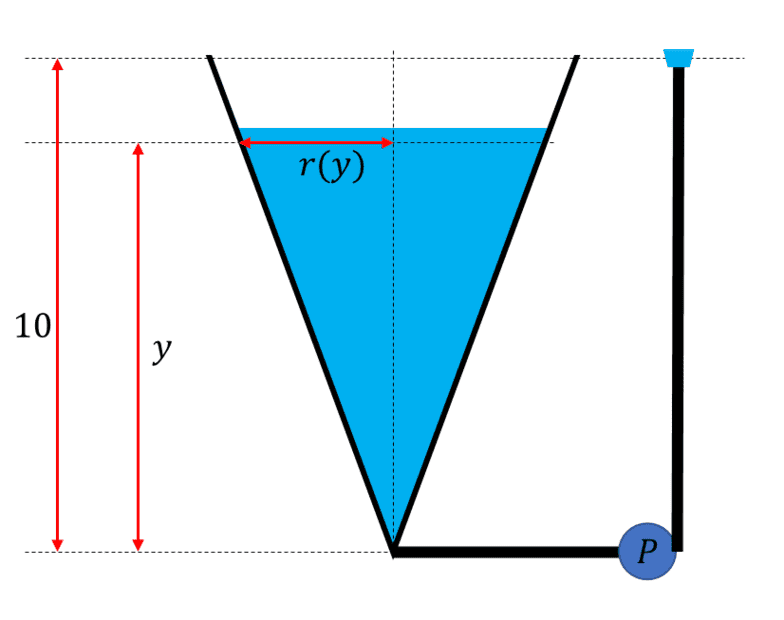# Work to empty a tank

songoku
Homework Statement:
A tank has the shape of an inverted circular cone with height 10 m and base radius 4 m. It is filled with water to a height of 8 m. Find the work required to empty the tank by pumping all of the water to the top of the tank. (The density of water is 1000 kg/m^3)
Relevant Equations:
W = ##\int F dx##
I take the origin to be at the apex of the cone. Using the similarity of the triangle, where ##r## is radius of water and ##y## is height of water from the apex of cone:

$$\frac{r}{y}=\frac{4}{10}$$
$$r=\frac{2}{5}y$$

The mass of water = ##\rho .V## = ##\rho . \pi r^2~\Delta y## = ##\rho . \pi \frac{4}{25}y^2~\Delta y##

The weight of water = ##\rho . \pi \frac{4}{25}y^2~\Delta y. g##

The distance needed to move the water to the top of the tank = 10 - y

The work needed:
$$W=\int_{2}^{10} \rho . \pi \frac{4}{25}y^2. g (10-y) ~dy$$

But I got wrong answer and the teacher said my origin was wrong. I still don't understand why I can't take origin to be at the apex.

Thanks

Homework Helper
Gold Member
Perhaps the issue is that you have not considered the surface of the water to be 8 m above the floor or bottom of the tank and 2 m below the apex?

I find this statement a little confusing: “empty the tank by pumping all of the water to the top of the tank.”

•songoku
Homework Helper
The distance needed to move the water to the top of the tank = 10 - y

That is what seems strange to me, not the choice of origin....##\ ##

•songoku
Homework Helper
2022 Award
If the apex is at the bottom, then the fluid occupies the region $0 \leq y \leq 8$, not the region $2 \leq y \leq 10$. If the apex is at the top, then the distance required to lift the fluid to the top of the tank is $y$, not $10 - y$.

•songoku
Homework Helper
So what's an inverted cone in the mind of the exercise composer ....
Since only the 4 m base radius is given , we may conclude that the top radius is 0 m and that some mind reading is required for this exercise##\ ##

•Lnewqban and songoku
Gold Member
So what's an inverted cone in the mind of the exercise composer ....
Since only the 4 m base radius is given , we may conclude that the top radius is 0 m and that some mind reading is required for this exercise##\ ##
Here is my interpretation, but I see your point.$$W_p = \int \Delta P ~dV\llap{-}$$

The change in pressure across the pump:

$$\Delta P = \rho g ( 10 - y )$$

The differential volume:

$$dV\llap{-} = \pi r^2 dy = \pi \left( \frac{2}{5} \right)^2 y^2 dy$$

$$W_p = \pi \rho g \left( \frac{2}{5} \right)^2 \int_{8}^{0} (10-y) y^2 dy$$

So as others have pointed out it stems from your limits of integration.

Last edited:
•Lnewqban and songoku
songoku
Ah, the limit of integration should be the region the fluid occupies. I thought it should be the distance moved by the fluids.

Thank you for the help and explanation Lnewqban, BvU, pasmith, erobz

•Lnewqban and erobz
Homework Helper
Gold Member
Another way to see it is the work or energy needed to move the whole mass of fluid between the height of its center of mass (full tank) and the height of the top of the tank.

•mathwonk, erobz and songoku
Homework Helper
Another way to see it is the work or energy needed to move the whole mass of fluid between the height of its center of mass (full tank) and the height of the top of the tank.
and if all is well, that should yield the same integral ...

##\ ##

•Lnewqban
Homework Helper
"Another way to see it is the work or energy needed to move the whole mass of fluid between the height of its center of mass (full tank) and the height of the top of the tank."

One reason I criticize recent "Thomas" calculus books, is to note that this simple and powerful principle is given in early editions of Thomas Calculus, (those written by Thomas himself), but not in later ones.

•Lnewqban
Mentor
@songoku, I'm hoping that you drew a sketch of the tank with the water level shown, similar to the one that @erobz provided. A cross-section of the tank would be sufficient. If you did draw such a sketch, you might have realized that the typical volume elements extend only between y = 0 and y = 8. Each layer has to be lifted from its position in the tank to a point 10 units above the bottom the tank.

In my experience teaching calculus for many years, I've found that very many students resist drawing a picture, thinking (mistakenly) that it will take too much time. It's better to take 3 minutes longer to get the right answer, than to skip this step and get the wrong answer.

•BvU, songoku, Lnewqban and 1 other person